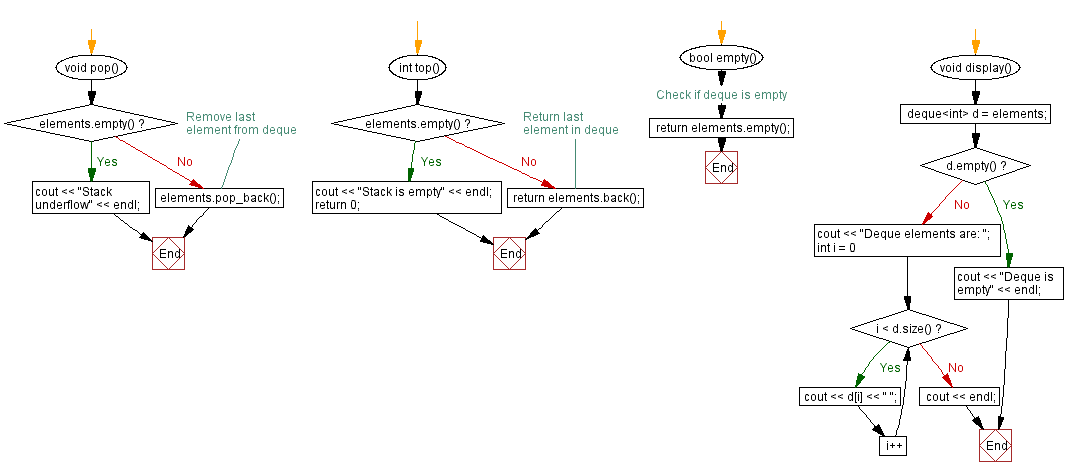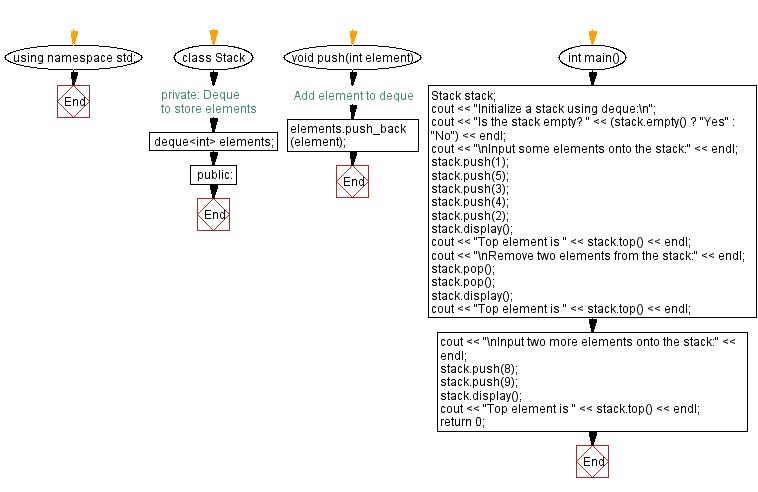﻿ C++ Implement a stack using a Deque with push, pop operations

# C++ Stack Exercises: Implement a stack using a Deque with push, pop operations

## C++ Stack: Exercise-28 with Solution

Write a C++ program to implement a stack using a Deque with push and pop operations. Check if the stack is empty or not and find the top element of the stack.

Test Data:
Initialize a stack using deque:
Is the stack empty? Yes
Input some elements onto the stack:
Deque elements are: 1 5 3 4 2
Top element is 2

Sample Solution:

C++ Code:

``````#include <iostream>
#include <deque>

using namespace std;

class Stack {
private:
deque<int> elements; // Deque to store elements

public:
void push(int element) {
elements.push_back(element); // Add element to deque
}

void pop() {
if (elements.empty()) {
cout << "Stack underflow" << endl;
} else {
elements.pop_back(); // Remove last element from deque
}
}

int top() {
if (elements.empty()) {
cout << "Stack is empty" << endl;
return 0;
} else {
return elements.back(); // Return last element in deque
}
}

bool empty() {
return elements.empty(); // Check if deque is empty
}

void display() {
deque<int> d = elements;
if (d.empty()) {
cout << "Deque is empty" << endl;
return;
}
cout << "Deque elements are: ";
for (int i = 0; i < d.size(); i++) {
cout << d[i] << " ";
}
cout << endl;
}

};
int main() {
Stack stack;
cout << "Initialize a stack using deque:\n";
cout << "Is the stack empty? " << (stack.empty() ? "Yes" : "No") << endl;
cout << "\nInput some elements onto the stack:" << endl;
stack.push(1);
stack.push(5);
stack.push(3);
stack.push(4);
stack.push(2);
stack.display();
cout << "Top element is " << stack.top() << endl;
cout << "\nRemove two elements from the stack:" << endl;
stack.pop();
stack.pop();
stack.display();
cout << "Top element is " << stack.top() << endl;
cout << "\nInput two more elements onto the stack:" << endl;
stack.push(8);
stack.push(9);
stack.display();
cout << "Top element is " << stack.top() << endl;
return 0;
}
``````

Sample Output:

```Initialize a stack using deque:
Is the stack empty? Yes

Input some elements onto the stack:
Deque elements are: 1 5 3 4 2
Top element is 2

Remove two elements from the stack:
Deque elements are: 1 5 3
Top element is 3

Input two more elements onto the stack:
Deque elements are: 1 5 3 8 9
Top element is 9
```

Flowchart:CPP Code Editor: